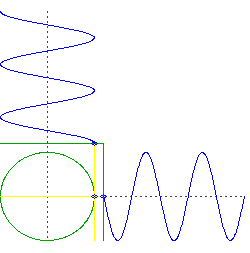# Two waves moving at right angles go in circles?

• B
Vriska
ylet x = Asinx, y = Acosx, apparently x^2 + y^2 = A^2 so this combination goes in circles. wot?

creating waves in a pool 90 degrees off and out of phase by 90 will make it move in circles? I'm skeptical, anyone have a video of an experiment that demonstrates this?

ylet x = Asinx, y = Acosx, apparently x^2 + y^2 = A^2 so this combination goes in circles. wot?creating waves in a pool 90 degrees off and out of phase by 90 will make it move in circles?
In a pool you have x,y,z not just x,y.

#### Attachments

•Vriska and anorlunda
Gold Member
will make it move in circles
What is it that you think will move in circles? The animation in your post just shows how two vectors which vary sinusoidally and are orthogonal in direction and phase (90°) have a resultant vector with a circular locus and the same angular frequency. That is not the same as two waves.
I can't be bothered to draw it out but the situation you are describing could be represented by a grid of horizontal lines sweeping upwards and a grid of vertical lines sweeping to the right. The resulting pattern would then be a square grid of peaks moving diagonally up and to the right, with troughs all round the peaks. (a moving waffle style of pattern). That would be the interference pattern due to two (equal amplitude and frequency) continuous waves moving at right angles to each other.
I can't think of a way to generate a wave that moves round in a circle unless you used a circular trough. (A guided wave)

Vriska
View attachment 215906

In a pool you have x,y,z not just x,y.
That's interesting, but now I'm wondering how on Earth do you get a straight line when the lines are in phase but perpendicular. Any insights on that?

thanks

Gold Member
Look for videos about Lissajous figures .

Vriska
What is it that you think will move in circles? The animation in your post just shows how two vectors which vary sinusoidally and are orthogonal in direction and phase (90°) have a resultant vector with a circular locus and the same angular frequency. That is not the same as two waves.
I can't be bothered to draw it out but the situation you are describing could be represented by a grid of horizontal lines sweeping upwards and a grid of vertical lines sweeping to the right. The resulting pattern would then be a square grid of peaks moving diagonally up and to the right, with troughs all round the peaks. (a moving waffle style of pattern). That would be the interference pattern due to two (equal amplitude and frequency) continuous waves moving at right angles to each other.
I can't think of a way to generate a wave that moves round in a circle unless you used a circular trough. (A guided wave)

That looks like the result when the waves are in phase ? these are out of phase, my equation and AT's gif suggest a circle i guess because x2+y^2 = a^2 thing

Perhaps two speakers spaced 90 to each other playing a sound out of phase would give you some circular droning maybe

Vriska
Look for videos about Lissajous figures .
Look for videos about Lissajous figures .

thank you so much! this is exactly what I was looking for, the pendulum experiment is particularly beautiful, I'm now completely convinced of the circle thing.

@sophiecentaur look at this!

Homework Helper
Gold Member
Nice video on Lissajous figures..

•Nidum
That's interesting, but now I'm wondering how on Earth do you get a straight line when the lines are in phase but perpendicular. Any insights on that?
When they are in phase then x=y which is obviously a 45° line.

Gold Member
That's interesting, but now I'm wondering how on Earth do you get a straight line when the lines are in phase but perpendicular. Any insights on that?

thanks
I suggest 'one thing at a time'.
Your question has to be either about Waves (which vary in time and space) or simple signals which vary in time; you should choose which you want to talk about. Lissajous Figures are not a Wave phenomenon - they are the result of adding time varying signals and the result is a time varying signal.
Two linear waves on a tank (as above), at right angles and in phase will produce a linear wave that travels diagonally. Two sinusoids, in quadrature, applied to the X and Y inputs of an oscilloscope will produce a circle (ellipse in the general case). If they are in phase, they will produce a diagonal line.
A simple Oscillation can be written Vt = V0 Cos(ωt)
There is no mention of position.
A simple wave, traveling along the x-axis can be written as
Vt,x = V0 Cos(ωt- kx)
See the difference?

•Vriska